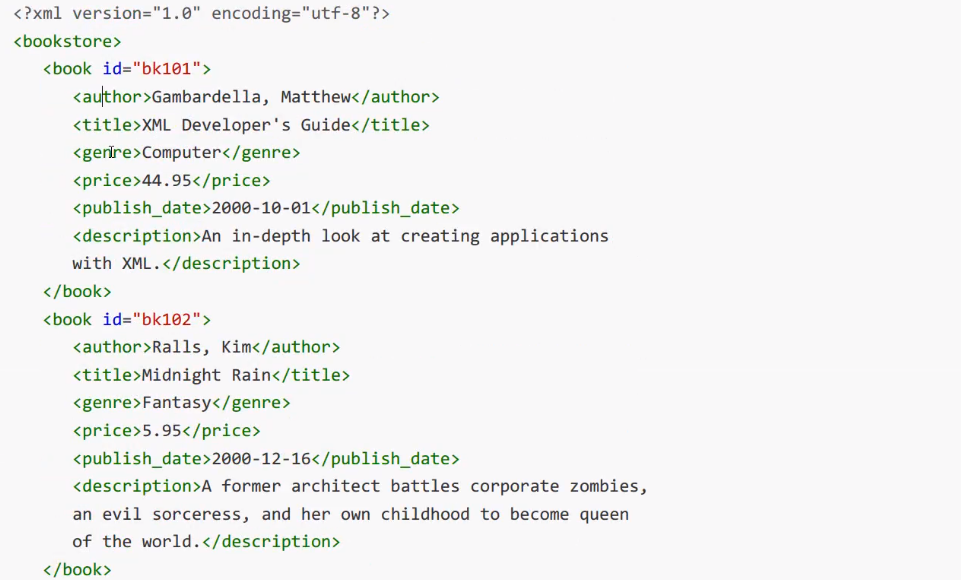# Python科学计算结果的存储与读取

+关注继续查看

Python科学计算结果的存储与读取

Python科学计算结果的存储与读取

# 一、前言

（1）并行多核计算简单，效率高

（2）占地不大

Matlab现在是越来越大了，您懂得……，但并卵，安装Anaconda也不到3GB吧？我添加了许多插件了的。

（3）通用性强

# 二、在Python中存储和读取科学计算结果

## 1、计算实例

 1 # 导入各种支持
2 import matplotlib.pyplot as plt
3 import numpy as np
4 import pandas as pd
5 import random
6 import math
7 import json
8 # import redis
9
10
11 # 建立科学计算
12 # Chebyshev map,初始值0。7，无关a值问题，注意取值是【-1，1】，可通过math.fabs实现切换m
13 def Chebyshev(maxIter, initial_value=0.7, a=1.0):
14 y = [0.0] * maxIter
15 for i in range(maxIter):
16 if i == 0:
17 y[i] = initial_value
18 else:
19 y[i] = math.cos(i / math.cos(y[i - 1]))
20 return y

## 2、采用Json存储和读取

# 采用json存储结果
def test_json_write():
maxIter = 500
chaos = Chebyshev(maxIter)
data = { 'chaos':chaos, 'maxIter':500}
datum = json.dumps(data, ensure_ascii=False)
with open('chaos.json', 'w') as f:
json.dump(data, f, indent=4, sort_keys=False)

# 采用json读取文件
fileName = "chaos"
with open('{}.json'.format(fileName), 'r') as fp:
chaos = result['chaos']
maxIter = result['maxIter']
plt.plot(chaos)
plt.show()

## 3、使用numpy的形式读取和使用

# 测试数组的存储方式
def test_numpy_save():
maxIter = 500
chaos = Chebyshev(maxIter)
data = np.array(chaos)
# data.tofile('chaos.npy',format='%e')
np.savez('chaos.npz', maxIter, chaos)

# 测试numpy文件的读取
# chaos=np.fromfile('chaos.npy',dtype=np.double)
chaos = result["arr_1"]
plt.plot(chaos)
plt.show()

# 三、总结

## 2、多个数组可使用词曲的方式存储62 0python爬虫实战实现XPath和lxml | python爬虫实战之六

1064 0[雪峰磁针石博客]python爬虫cookbook1爬虫入门
1970 0Python爬虫实战示例-51job和豆瓣电影
2018年7月16日笔记 1.conda常用命令 1.1 列出当前环境的所有库 命令：conda list 在cmd中运行命令如下图所示： 图片.png-36.
1356 02921 01432 0python 爬虫入门之正则表达式 一
1092 0Python爬虫：爬取资源站点列表
766 0python爬虫入门基本知识
3007 0Python爬虫入门一之综述

3172 0+关注

393

9